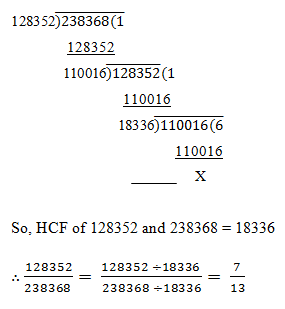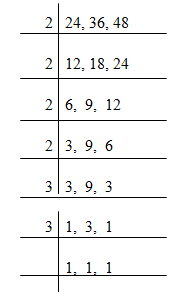# HCF and LCM ( 6 to 10 )

#### PREVNEXT

HCF and LCM ( 6 to 10 ):

# APTITUDE QUESTIONS (HCF AND LCM):

6. Reduce 128352/238368 to its lowest terms.

A)    3/4

B)    5/13

C)    7/13

D)    9/13

E)    None of these

Explanation:7. The sum of two numbers is 512 and their HCF is 32. How many numbers of pairs will satisfy the above condition?

A)    5

B)    4

C)    8

D)    12

E)    None of these

Explanation:

Let the required numbers be 32a and 32b. Then, 32a + 32b = 512

• 32 (a + b) = 512
• a + b = 512/32
• a + b = 16

Now, co-primes with sum 16 are (1, 15), (3, 13), (5, 11) and (7, 9).

∴ required numbers are (32 * 1, 32 * 15), (32 * 3, 32 * 13), (32 * 5, 32 * 11), (32 * 7, 32 * 9).

The number of such pairs is 4.

8. If the ratio of two numbers is 4:5 and their HCF is 5 than what will be the LCM?

A)    10

B)    25

C)    50

D)    100

E)    None of these

Explanation:

Let the numbers be 4x and 5x. Then, their HCF is = x.

So, x = 5.

So, the numbers are 20 and 25.

LCM of 20 and 25 = 100

9. Find the lowest common multiple of 24, 36 and 48.

A)    120

B)    240

C)    360

D)    144

E)    None of these

Explanation:LCM = 2 * 2 * 2 * 2 * 3 * 3 = 144

10. The product of two numbers is 2028 and their HCF is 13. The number of such pairs is:

A)    6

B)    5

C)    3

D)    2

E)    None of these

Explanation:

Let the numbers be 13a and 13b. Then, 13a * 13b = 2028

• ab = 2028/169
• ab = 12

The product of two numbers is 12 and its co-prime numbers are (1, 12) and (3, 4)

So, the required numbers are (13 * 1, 13 * 12), (13 * 3, 13 * 4).

So, there are 2 such pairs.